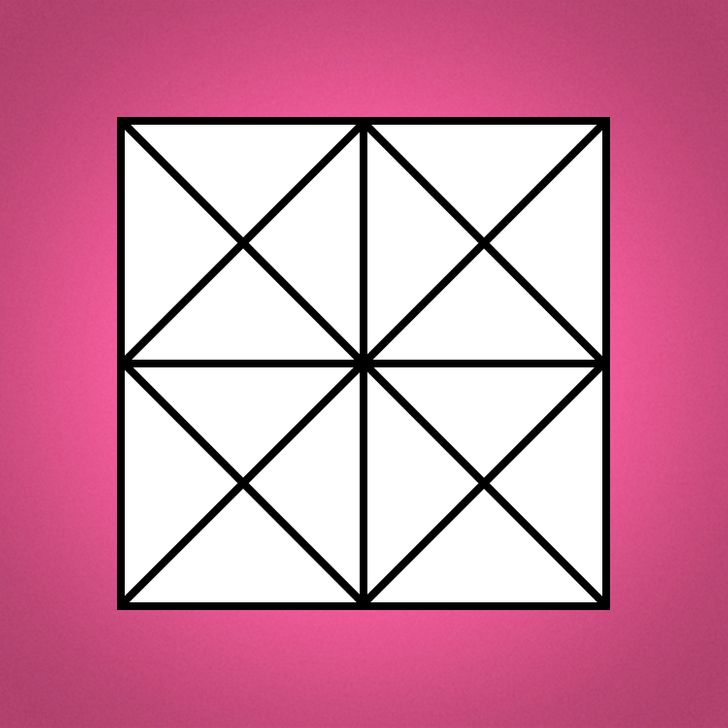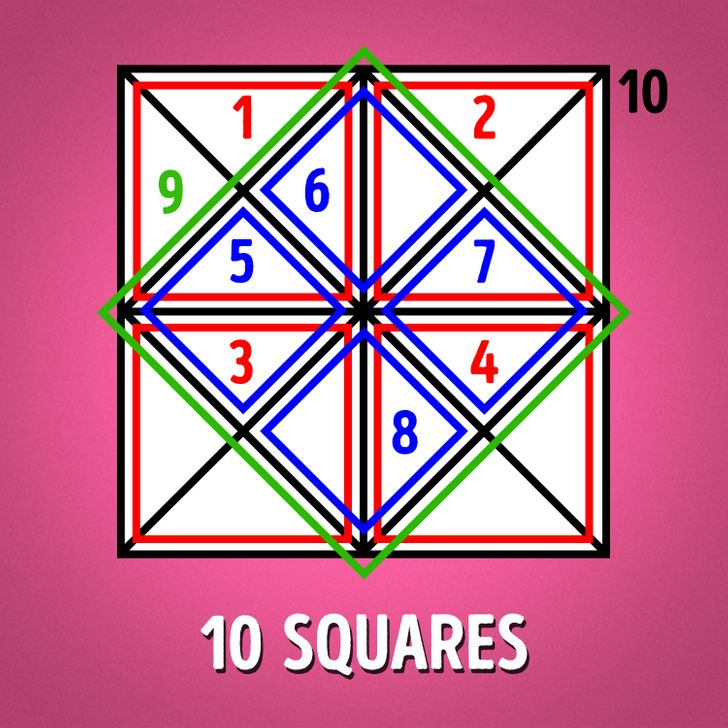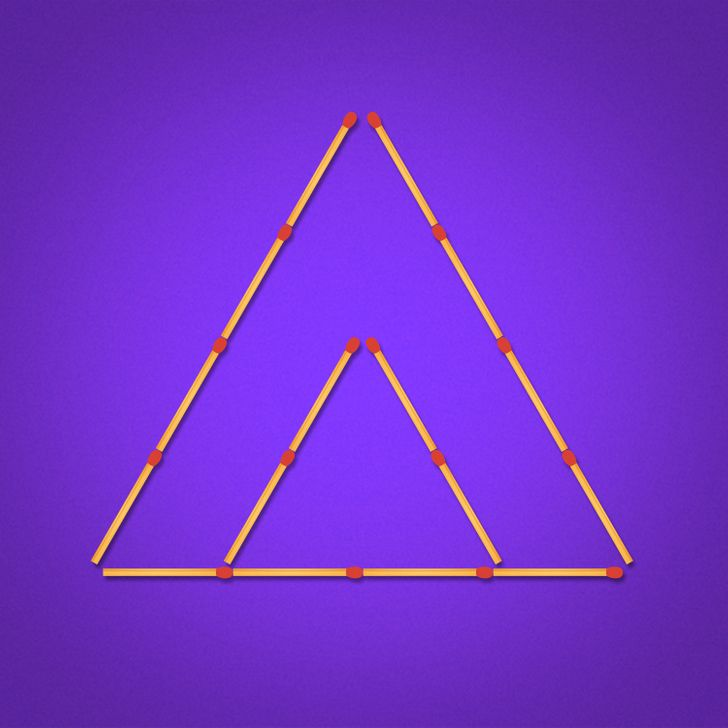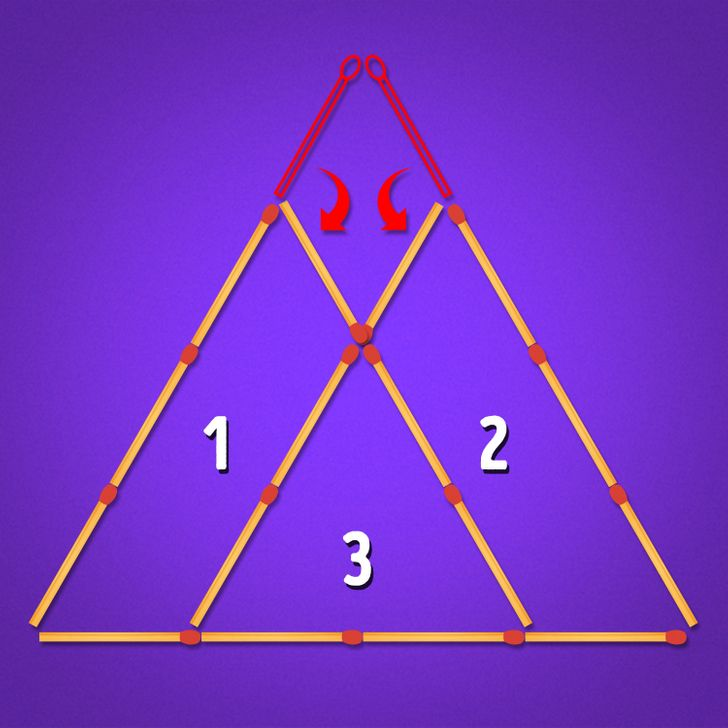# 10 Brain-Teasers That Every Riddle Pro Can Solve in One Minute

It’s always fun to solve brain-teasers with a group of friends. So, get together and give it a go. You’ll need to crack these 10 riddles in just one minute and then compare the results. In this particular case, quality is just as important as quantity, in other words, the winner is the one who gives the most correct answers in the shortest time.

Just tap on the picture to see the answer.

We at Bright Side found a few brain teasing riddles for our readers. Get your timer ready, and remember that you only have one minute.

### 1. How many squares can you find?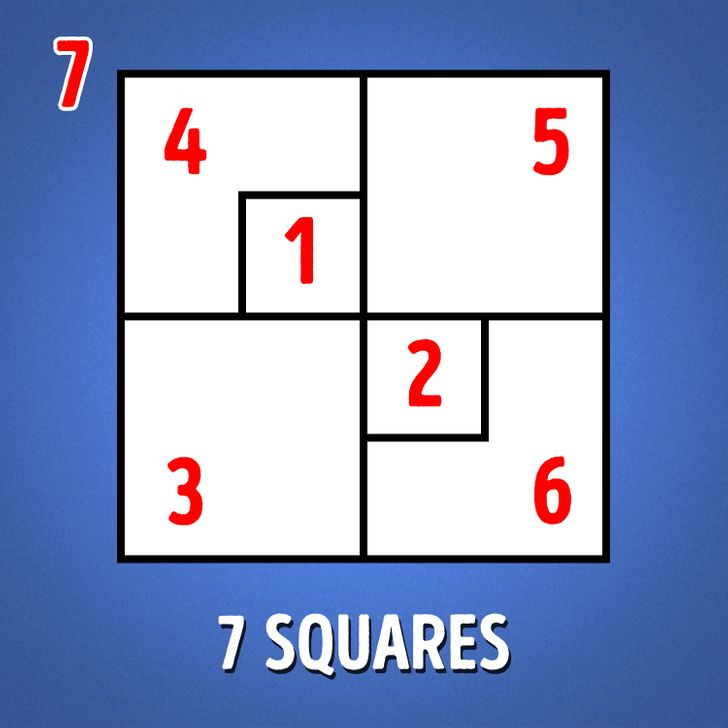### 2. How many pentagons can you count?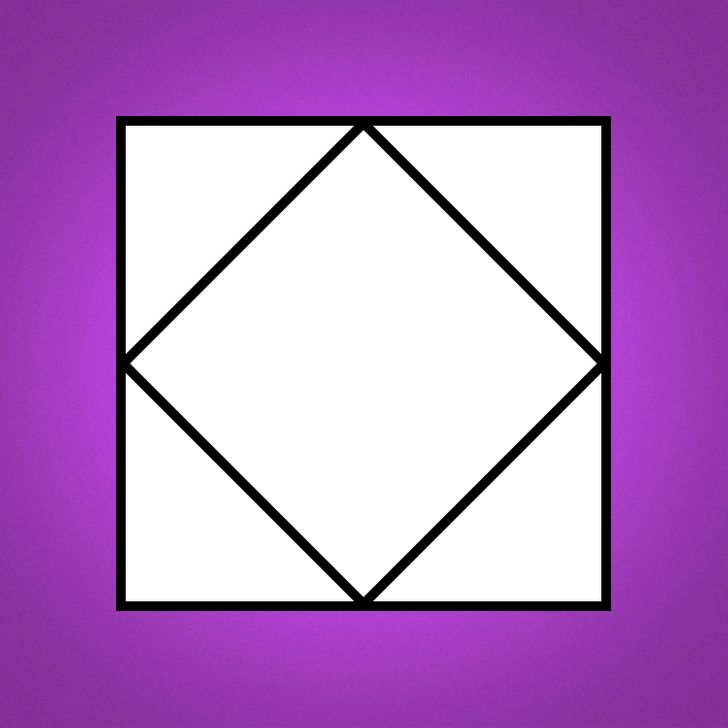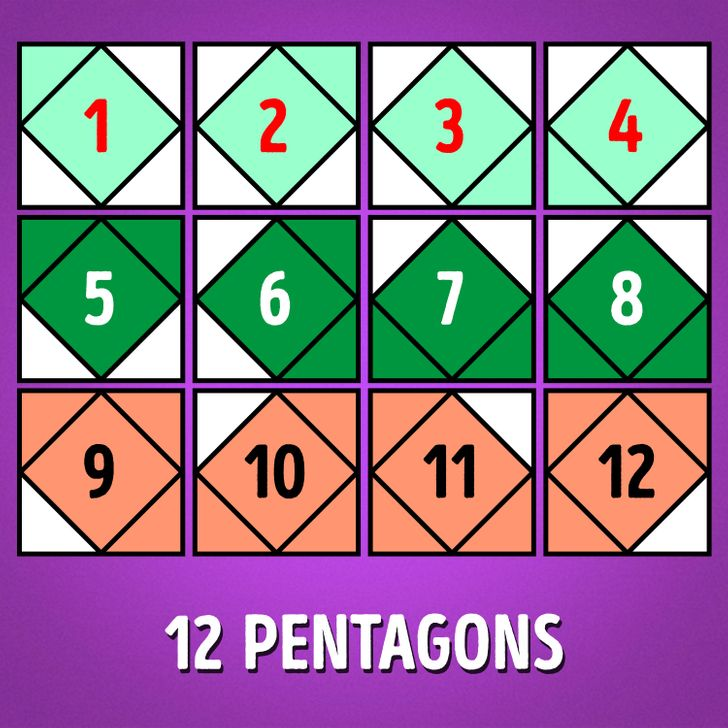### 3. Move 3 matchsticks to get 4 equal triangles.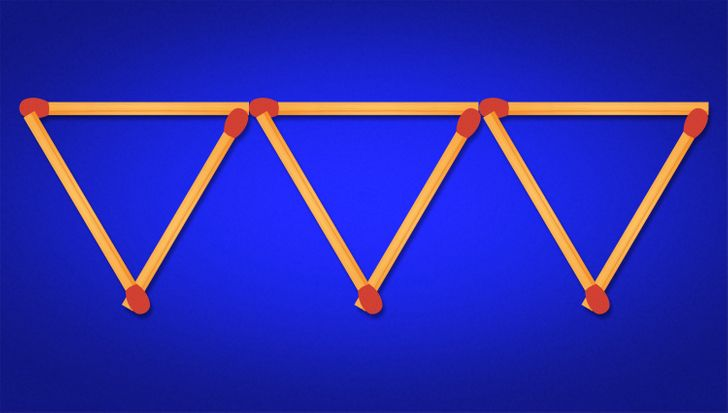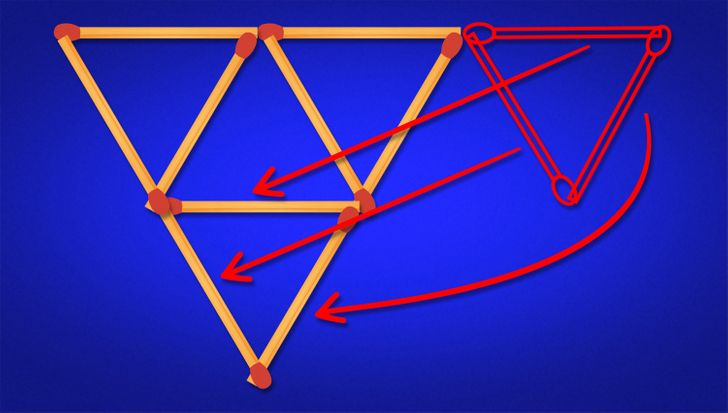### 4. Find 2 ways to get a square by moving 2 matchsticks.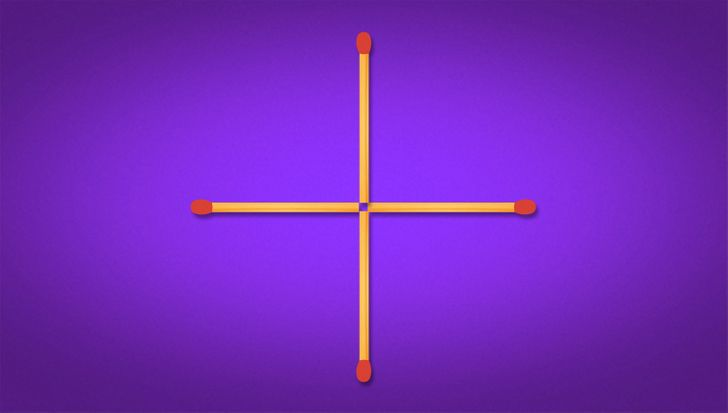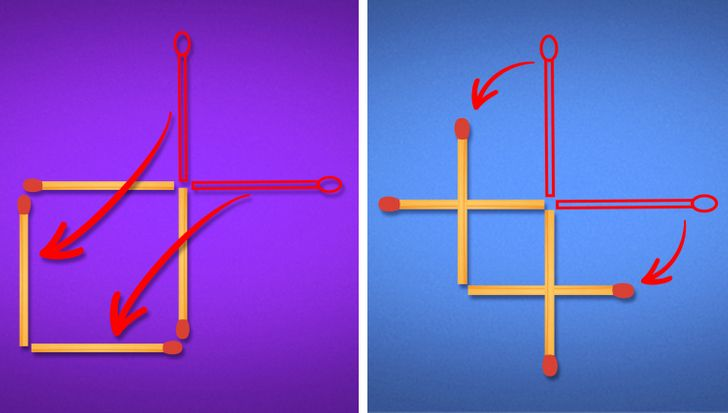### 5. How many squares can you find?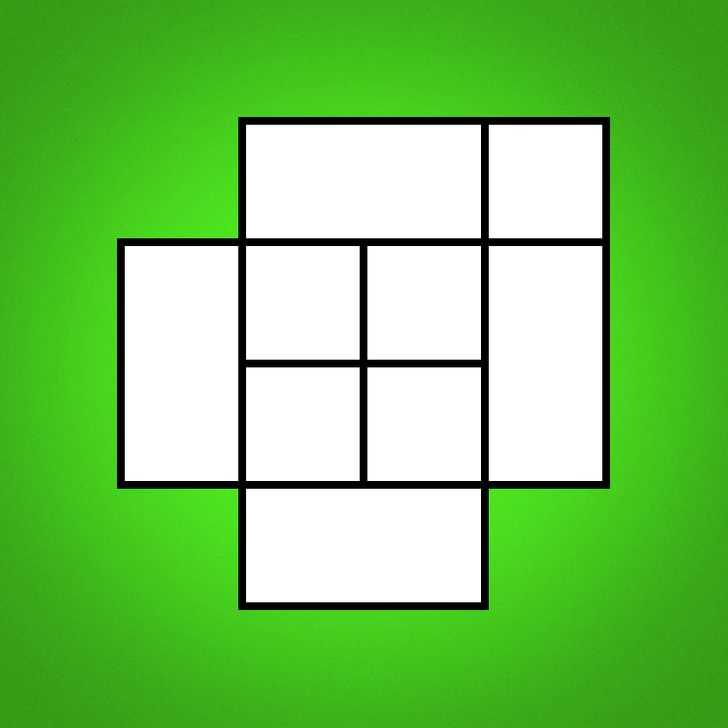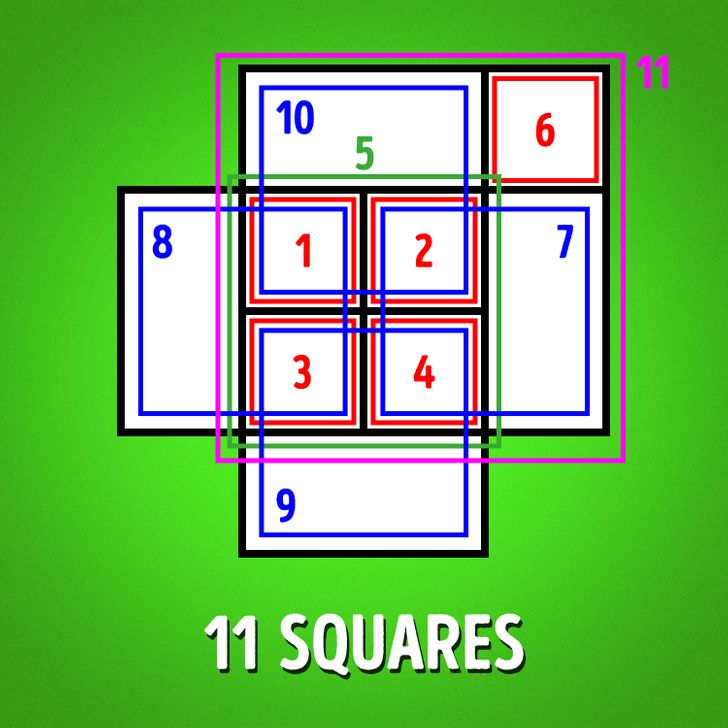### 6. How many triangles can you find?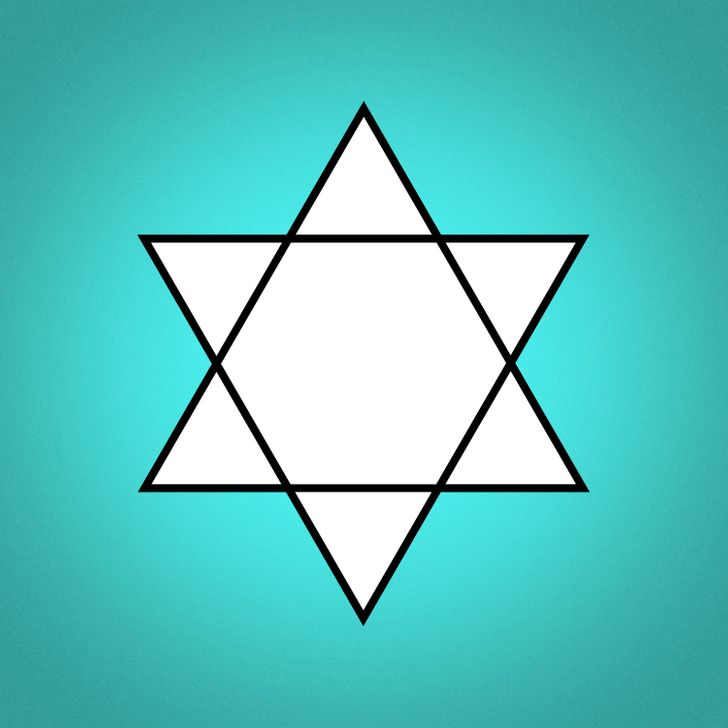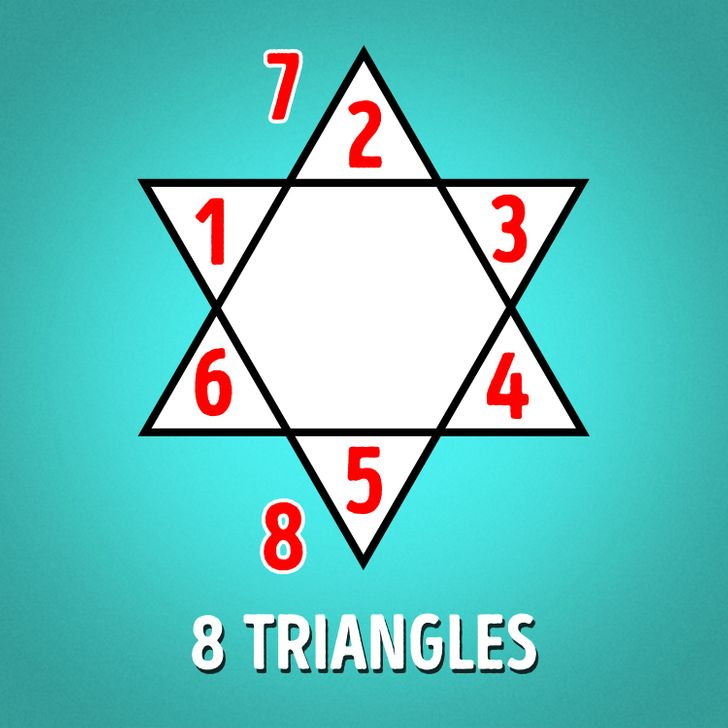### 7. How many squares can you find?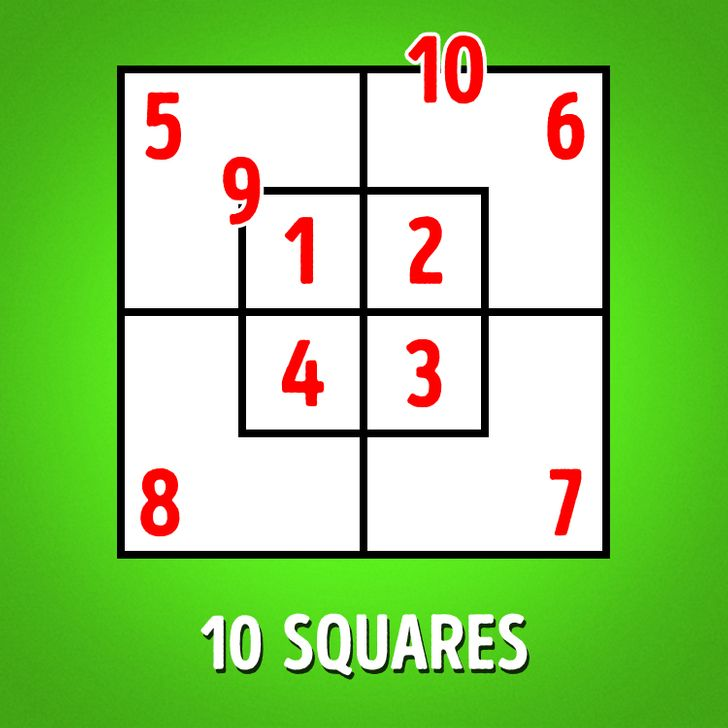### 8. How many triangles can you spot?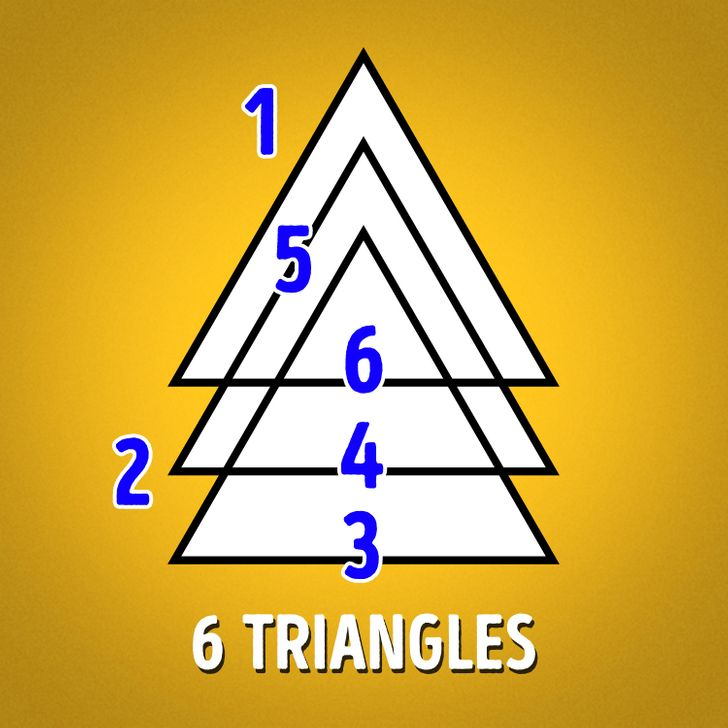### 9. How many squares can you see?